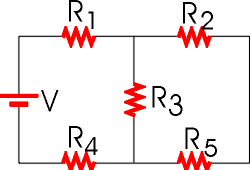# Problem: Find the equivalent resistance of the circuit shown in the figure. Assume that V = 14.5 V, R1 = 9.86 Ω, R2 = 3.25 Ω, R3 = 3.93 Ω, R4 = 3.86 Ω and R5 = 7.24 Ω.

###### FREE Expert Solution

Equivalent resistance for 2 resistors in parallel:

$\overline{){{\mathbf{R}}}_{{\mathbf{eq}}}{\mathbf{=}}\frac{{\mathbf{R}}_{\mathbf{1}}{\mathbf{R}}_{\mathbf{2}}}{{\mathbf{R}}_{\mathbf{1}}\mathbf{+}{\mathbf{R}}_{\mathbf{2}}}}$

Equivalent resistance for resistors in series:

$\overline{){{\mathbf{R}}}_{{\mathbf{eq}}}{\mathbf{=}}{{\mathbf{R}}}_{{\mathbf{1}}}{\mathbf{+}}{{\mathbf{R}}}_{{\mathbf{2}}}{\mathbf{+}}{\mathbf{.}}{\mathbf{.}}{\mathbf{.}}{\mathbf{+}}{{\mathbf{R}}}_{{\mathbf{n}}}}$

R2 and R5 are in series.###### Problem DetailsFind the equivalent resistance of the circuit shown in the figure. Assume that V = 14.5 V, R1 = 9.86 Ω, R2 = 3.25 Ω, R3 = 3.93 Ω, R4 = 3.86 Ω and R5 = 7.24 Ω.